# parallelogram theorem

## POLYGONAL REGION

Polygon region can be expressed as the union of a finite number of triangular regions in a plane such that if two of these intersect, their intersection is either a point or a line segment. It is the shaded portion including its sides as shown in the figure.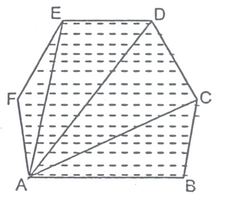### (a) Area Axioms:

Every polygonal region R has an area, measure in square unit and denoted by ar(R).

• (i) Congruent area axiom :if R1 and R2 be two regions such that R1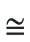R2 then ar(R1) = ar (R2).
• (ii) Area monotone axiom :If R1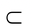R2, then are (R1)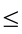ar(R2).
• (iii) Area addition axiom :If R1 are two polygonal regions, whose intersection is a finite number of points and line segments and R = R1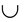R2, then ar (R) = ar(R1) + ar(R2).
• (iv) Rectangular area axiom :If AB = a metre and AD = b metre then, ar (Rectangular region ABCD) = ab sq. m.

### (b) Unit of Area :

There is a standard square region of side 1 metre, called a square metre, which is the unit of area measure. The area of a polygonal region is square metres (sq. m or m2) is a positive real number

### AREA ADDITION AXIOM:

Total area R of the plane figure ABCD is the sum of two polygonal regions R1 and R2, i.e. ar. (R) = ar.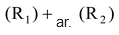### THEOREM 1

A diagonal of parallelogram divides it into two triangles of equal area.

Given: A parallelogram ABCD whose one of the diagonals is BD.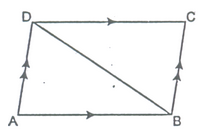To prove: ar (ΔABD) = ar (ΔCDB).

Proof: In ΔABD and ΔCDB.

AB = DC [Opp. sides of a ||gm]

AD = BC [Opp. sides of a ||gm]

BD = BD [Common side]

∴ ΔABD≅ΔCDB [By SSS]

∴ ar (ΔABD) = ar(ΔCDB) [Congruent area axiom] Hence Proved.

### THEOREM 2

Prove that parallelogram on the same base and between same parallel are equal in area.

Given: Parallelogram ABCD and ABEF are on the same base AB and between the same parallels AB and CF.

To Prove: ar. (ABCD) = ar. (ABEF)

Proof: In ΔADF and ΔBCE, we have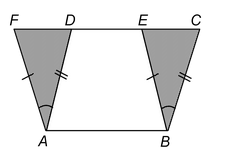AF = BE [Opposite sides of the ||gms]

∠AFD = ∠BEC [Corresponding angles]

∠ADF = ∠BCE [Corresponding angles]

∴ ΔADF≅ΔBCE [By AAS Congruence]

⇒ ar. (ΔADF) = ar. (ΔBCE) [Congruence area axiom]

Adding ar.(quadrilateral ABED) to both sides, we get

ar. (ΔADF) + ar.(quadrilateral ABED) = ar (ΔBCE) + ar.(quadrilateral ABED)

⇒ ar. ||gmABEF = ar. ||gmABCD [Area addition axiom]

### THEOREM 3

Prove that parallelogram and a rectangle on the same base and between the same parallels are equal in area.

Given :A Parallelogram ABCD and a rectangle ABML are on the same base AB and between the same parallels AB and CL.

To Prove : ar. ||gmABCD = ar. rectangle ABML.

Proof :In ΔADL and ΔBCM, we have

AL = BM [Opposite sides of a rectangle]

∠ALD =∠BMC[Corresponding angles]

∠ADL = ∠BCM  [Corresponding angles]

∴ ΔADL≅ΔBCM [By AAS congruence]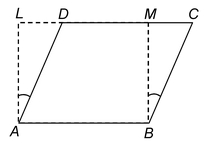⇒ ar. (ΔADL) = ar. (ΔBCM)

Adding ar. (quadrilateral ABMD) to both sides, we get

ar. ΔADL + ar. quadrilateral ABMD = ar. ΔBCM + ar. quadrilateral ABMD

or ar. of rectangle ABML = ar. of ||gmABCD.

### THEOREM 4

If a triangle and a parallelogram are on the same base and between the same parallels, then prove that the area of the triangle is equal to half the area of the parallelogram.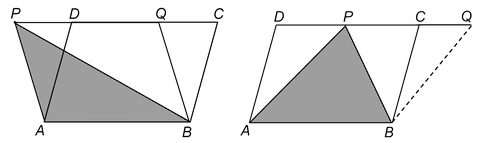(i) (ii)

Given: AParallelogram ABCD and a triangle APB are on the same base AB and between the same parallels AB and PC.

To Prove: ar.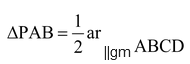Const: Through B draw BQ || AP which meets PC or PC produced at Q. Join BQ

Proof: Since the parallelogram ABQP and the parallelogram ABCD stand on the same base AB and between same parallels AB and PQ or DQ.

∴ ar. ||gmABQP = ar. ||gmABCD [By theorem 1] …(i)

Since the diagonal of a ||gmABQP divides it into two Δs of equal area

∴ ar. ||gmABQP = 2 ar. (ΔPAB) …(ii)

From (i) and (ii), we have

2 ar. (ΔPAB) = ar. ||gmABCD

⇒ ar..

### THEOREM 5

The arc of parallelogram is the product of its base and the corresponding altitude.

Given: A||gm ABCD in which AB is the base and AL is the corresponding height.

To prove: Area (||gmABCD) = AB × AL.

Construction: Draw BM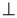DC so that rectangle ABML is formed.

Proof: ||gm ABCD and rectangle ABML are on the same base AB and between the same parallel lines AB and LC.

∴ ar(||gmABCD) = ar(rectangle ABML) = AB × AL.

∴ area of a ||gm = base × height.

Ex: Prove that a diagonal of a parallelogram divides it into two triangles of equal area.

Solution: Given: A parallelogram ABCD in which BD is one of the diagonals.

To prove  → ar((ΔABD) = ar((ΔCDB)

Proof: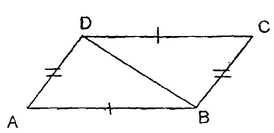In triangles ABD and CDB, we have

AB = CD (in a parallelogram opposite sides are of equal length)

and BD = DB (common side)

⇒ΔABD (ΔCDB (by SSS)

Hence by congruent area axiom ar((ΔABD) = ar((ΔCDB).

Ex. A parallelogram ABCD and a rectangle ABPQ are on the same base AB and between the same parallels AB and CQ. If AB = 8 cm and AQ = 6 cm, find the area of ||gmABCD.

Sol. A rectangle is a parallelogram in which each angle is 90° and parallelogram on the same base and between same parallels are equal in area.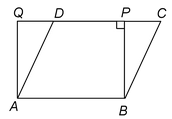∴ ar. (||gmABCD) = ar. (rectangle ABPQ)

But ar. (||gmABPQ) = AB×AQ

= (8 × 6) cm2 = 48 cm2

∴ Area of parallelogram ABCD = 48 cm2.

Ex. In a parallelogram ABCD, AB = 8 cm. The altitudes corresponding to sides AB and AD are respectively 4 m and 5 cm. Find AD.

Sol. We know that, Area of a parallelogram = Base × Corresponding altitude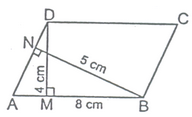∴ Area of parallelogram ABCD = AD × BN = AB × DM

⇒ AD × 5 = 8 × 4

⇒ AD =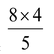= 6.4 cm. Ans.

Ex. In figure, ABCD is a parallelogram, AE⊥CD and CF⊥AD. If AB = 16 cm, AE = 8 cm and CF = 10 cm, find AD.

Sol. AB = 16 cm, AE = 8 cm and CF = 10 cm, AD= ?

ar. ||gmABCD (on base AD) = ar. ||gmABCD (on base CD)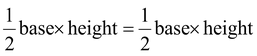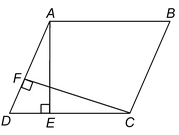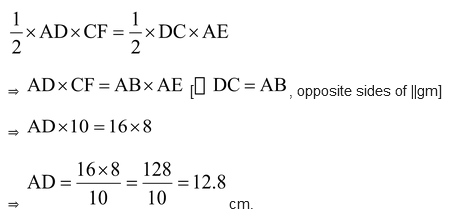Ex: ABCD is a quadrilateral and BD is one its diagonals as shown in figure. Show that ABCD is a parallelogram and find its area.

Sol: In ΔABD and ΔCDB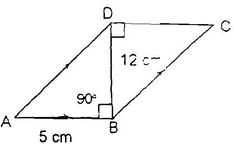∠ABD = ∠CBD = 90o (alternate interior angles are equal)

∠BDA = ∠BDC

Hence, ABCD is a parallelogram.

Now, area of parallelogram ABCD = (base ×corresponding altitude)

⇒area of parallelogram ABCD = AB × BD = 5 ×12 sq. units = 60 sq. cms.

Ex: Prove that parallelograms on the same base and between the same parallel lines are equal in area.

Sol: Given: Two parallelogram ABCD and PBCQ having same base BC and between the same parallel lines BC and AQ.

To Prove: ar(parallelogram ABCD)= ar (parallelogram PBCQ)

Proof: In triangles ABP and DCQ,

∠BAP = ∠CDQ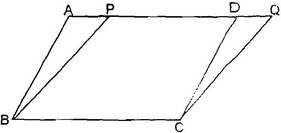(Corresponding angles when AQ intersects parallel lines AB and DC).

∠BPA = ∠CDQ

(Corresponding angles when AQ intersects parallel lines BP and CQ).

AB = DC (Opposite sides of a parallelogram)

∴ΔABP ≅ ΔDCQ (AAS)

Hence area ΔABP = area ΔDCQ

or, area ΔABP + area BPDC = area ΔDCQ + area Δ BPDC

∴ar (parallelogram ABCD) = ar (parallelogram PBCQ).